## ML Aggarwal Class 8 Solutions for ICSE Maths Chapter 2 Exponents and Powers Ex 2.2

Question 1.
Express the following numbers in standard form:
(i) 0.0000000000085
(ii) 0.000000000000942
(iii) 6020000000000000
(iv) 0.00000000837
Solution:Question 2.
Express the following numbers in the usual form:
(i) 3.02 × 10-6
(ii) 1-007 × 1011
(iii) 5.375 × 1014
(iv) 7.579 × 10-14
Solution: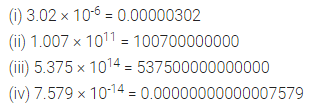Question 3.
Express the number appearing in the following statements in standard form:
(i) The mass of a proton is 0.000000000000000000000001673 gram.
(ii) The thickness of a piece of paper is 0.0016 cm.
(iii) The diameter of a wire on a computer chip is 0.000003 m.
(iv) A helium atom has a diameter of $$\frac { 22 }{ 100000000000 }$$ m
(v) Mass of a molecule of hydrogen gas is about 0.00000000000000000000334 tons.
(vi) The human body has 1 trillion cells which vary in shapes and sizes.
(vii) The distance from the Earth of the Sun is 149,600,000,000 m.
(viii) The speed of light is 300,000,000 m/sec.
(ix) Mass of the Earth is 5,970,000,000,000,000,000,000,000 kg.
(x) Express 3 years in seconds.
(xi) Express 7 hectares in cm2.
(xii) A sugar factory has annual sales of 3 billion 720 million kilograms of sugar.
Solution: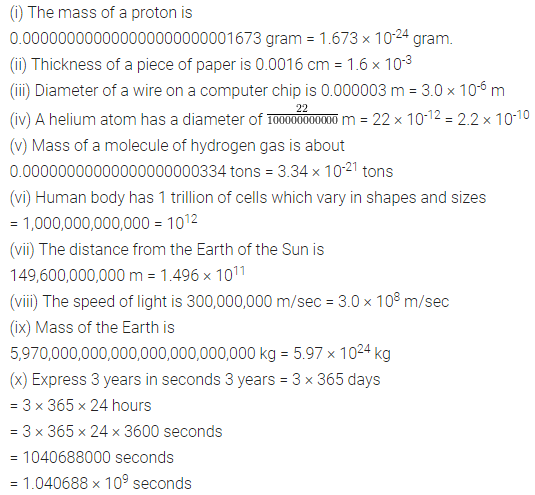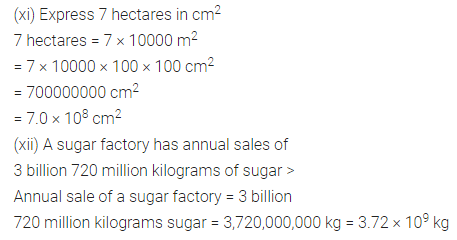Question 4.
Compare the following:
(i) Size of a plant cell to the thickness of a piece of paper.
(ii) Size of a plant cell to the diameter of a wire on a computer chip.
(iii) The thickness of a piece of paper to the diameter of a wire on a computer chip.
Given size of plant cell = 0.00001275 m
Thickness of a piece of paper = 0.0016 cm
Diameter of a wire on a computer chip = 0.000003 m
Solution: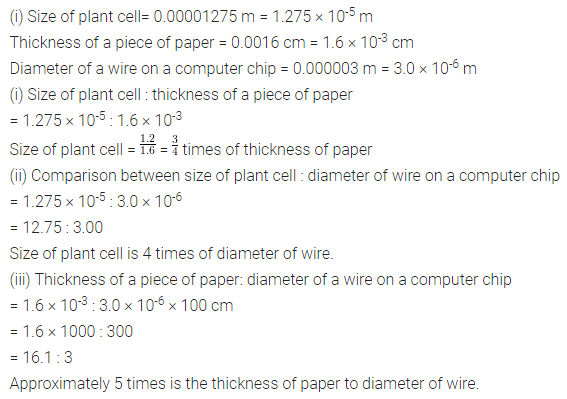Question 5.
The number of red blood cells per cubic millimetre of blood is approximately 5.5 million. If the average body contains 5 litres of blood, what is the total number of red cell in the body? (1 litre = 1,00,000 mm3)
Solution: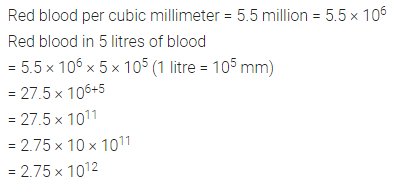Question 6.
Mass of Mars is 6.42 × 1029 kg and the mass of the sun is 1.99 × 1030 kg. What is the total mass?
Solution: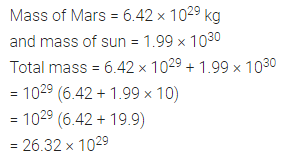Question 7.
A particular star is at a distance of about 8.1 × 1013 km from the Earth. Assuming that the light travels at 3 × 108 m/sec, find how long does light take from that star to reach the Earth.
Solution: